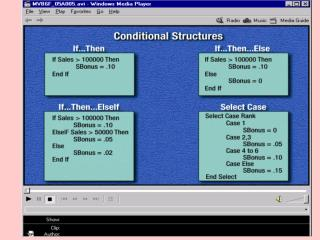DownloadDownload PresentationLooping Structures

# Looping Structures

Télécharger la présentation## Looping Structures

- - - - - - - - - - - - - - - - - - - - - - - - - - - E N D - - - - - - - - - - - - - - - - - - - - - - - - - - -
##### Presentation Transcript

1. Looping Structures Do Loops, For Next

2. Do • strPassword = InputBox("Enter your password") • Loop While strPassword <> "Lefty" Do...Loop While structures check the condition after executing the code and repeat a code block until the test expression evaluates as false. • The example code will loop until the correct password is entered

3. Dim intLoopCount As Integer • Do • intLoopCount = intLoopCount + 1 • Loop Until MsgBox("Loop?", vbYesNo) = vbNo Do...Loop Until structures check the condition after executing the code and repeat a code block until the test expression evaluates as true. • The example code will loop until the No button is clicked in a message box.

4. Dim strPasswordTry As String • Const PASSWORD As String = "password" • Do While strPasswordTry <> PASSWORD • strPasswordTry = InputBox("Enter password") • Loop Do While...Loop structures check the condition before executing the code. The code in the loop is executed only if the condition evaluates as true, and repeats until the test expression evaluates as false. • The example code will ask the user for a password until the correct password is typed into the input box

5. Dim intValue As Integer • Dim strInput As String • Do Until intValue = 3 • strInput = InputBox("Pick a number between 1 and 5") • intValue = Val(strInput) • Loop Do Until...Loop structures check the condition before executing the code. The code in the loop is executed only if the condition evaluates as false, and repeats until the test expression evaluates as true.

6. Dim strMsg As String • Dim strFilespec As String • Dim strMatch As String • strMsg = "Enter a file specification." • 'Get file extension • strFilespec = InputBox(strMsg) • 'Find first match • strMatch = Dir(strFilespec) • Do Until Len(strMatch) = 0 • 'Display matching files • MsgBox strMatch • 'Find next match • strMatch = Dir() • Loop • MsgBox "There are no more matching files." CHALLENGE:

7. Forcounter = startToend[Step increment] • [statements] • Next[counter] • When both the condition and the number of times a code • block will execute is known, use a For loop.

8. Dim intNumStudents As Integer • Dim intCounter As Integer • Dim sglScore As Single • Dim sglTotalScore As Single • Dim sglAverage As Single • intNumStudents = InputBox(prompt:="How many students?") • For intCounter = 1 To intNumStudents • sglScore = CSng(Val(InputBox(prompt:="Enter score"))) • sglTotalScore = sglTotalScore + sglScore • Next intCounter • sglAverage = sglTotalScore / intNumStudents • The following example code displays an input box that prompts the user to enter a number. It then executes • the code in the For...Next loop the specified number of times, calculating and displaying a result.

9. Dim intOuter As Integer, intInner As Integer • Dim strOut As String • ' Outer Loop • For intOuter = 1 To 10 • ' Inner Loop • For intInner = 1 To 10 • ' Concatenate Outer * Inner results to strOut • strOut = strOut & " " & Format(intOuter * intInner, "@@@") • Next intInner • ' Add a carriage return and linefeed to end of line • strOut = strOut & vbCrLf • Next intOuter • ' Monospaced font • Me.Font = "Courier New" • ' Display strOut on form • Me.Print strOut • The first For...Next loop generates each new line within the table. The second • For...Nextloop is nested within the first and generates each column within a row